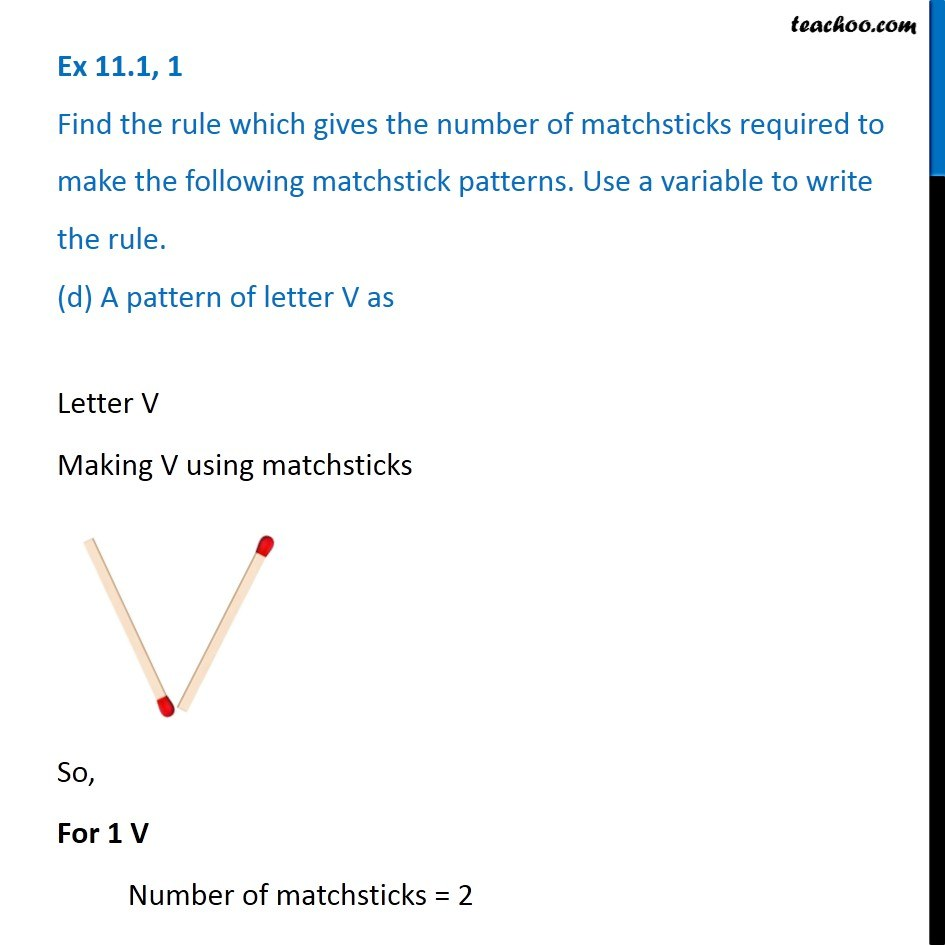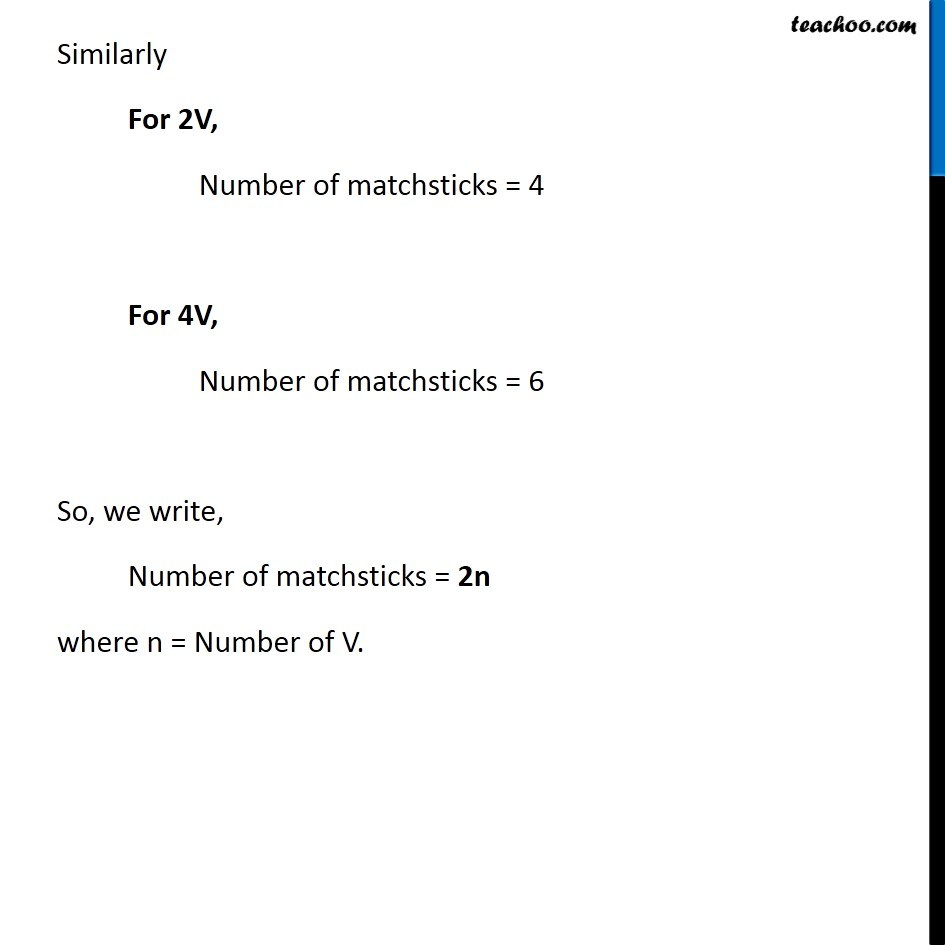Ex 11.1

Chapter 11 Class 6 Algebra
Serial order wiseLearn in your speed, with individual attention - Teachoo Maths 1-on-1 Class

### Transcript

Ex 11.1, 1 Find the rule which gives the number of matchsticks required to make the following matchstick patterns. Use a variable to write the rule. (d) A pattern of letter V asLetter V Making V using matchsticks So, For 1 V Number of matchsticks = 2 Similarly For 2V, Number of matchsticks = 4 For 4V, Number of matchsticks = 6 So, we write, Number of matchsticks = 2n where n = Number of V.